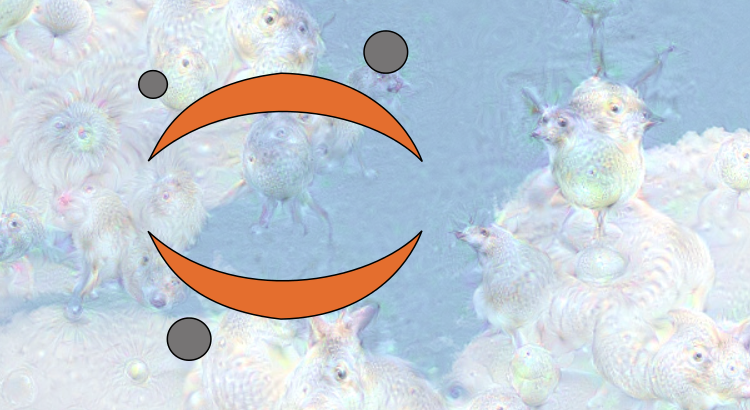## Hyperparameter sample-size dependence

Here, we briefly review a subtlety associated with machine-learning model selection: the fact that the optimal hyperparameters for a model can vary with training set size, $N.$ To illustrate this point, we derive expressions for the optimal strength for both $L_1$ and $L_2$ regularization in single-variable models. We find that the optimal $L_2$ approaches a finite constant as $N$ increases, but that the optimal $L_1$ decays exponentially fast with $N.$ Sensitive dependence on $N$ such as this should be carefully extrapolated out when optimizing mission-critical models.

## Interpreting the results of linear regression

Our last post showed how to obtain the least-squares solution for linear regression and discussed the idea of sampling variability in the best estimates for the coefficients. In this post, we continue the discussion about uncertainty in linear regression — both in the estimates of individual linear regression coefficients and the quality of the overall fit.

Specifically, we’ll discuss how to calculate the 95% confidence intervals and p-values from hypothesis tests that are output by many statistical packages like python’s statsmodels or R. An example with code is provided at the end.## Linear Regression

We review classical linear regression using vector-matrix notation. In particular, we derive a) the least-squares solution, b) the fit’s coefficient covariance matrix — showing that the coefficient estimates are most precise along directions that have been sampled over a large range of values (the high variance directions, a la PCA), and c) an unbiased estimate for the underlying sample variance (assuming normal sample variance in this last case). We then review how these last two results can be used to provide confidence intervals / hypothesis tests for the coefficient estimates. Finally, we show that similar results follow from a Bayesian approach.

Last edited July 23, 2016.## Average queue wait times with random arrivals

Queries ping a certain computer server at random times, on average $\lambda$ arriving per second. The server can respond to one per second and those that can’t be serviced immediately are queued up. What is the average wait time per query? Clearly if $\lambda \ll 1$, the average wait time is zero. But if $\lambda > 1$, the queue grows indefinitely and the answer is infinity! Here, we give a simple derivation of the general result — (9) below.## Improved Bonferroni correction factors for multiple pairwise comparisons

A common task in applied statistics is the pairwise comparison of the responses of $N$ treatment groups in some statistical test — the goal being to decide which pairs exhibit differences that are statistically significant. Now, because there is one comparison being made for each pairing, a naive application of the Bonferroni correction analysis suggests that one should set the individual pairwise test sizes to $\alpha_i \to \alpha_f/{N \choose 2}$ in order to obtain a desired family-wise type 1 error rate of $\alpha_f$. Indeed, this solution is suggested by many texts. However, implicit in the Bonferroni analysis is the assumption that the comparisons being made are each mutually independent. This is not the case here, and we show that as a consequence the naive approach often returns type 1 error rates far from those desired. We provide adjusted formulas that allow for error-free Bonferroni-like corrections to be made.

[edit (7/4/2016): After posting this article, I’ve since found that the method we suggest here is related to / is a generalization of Tukey’s range test — see here.]

[edit (6/11/2018): I’ve added the notebook used below to our Github, here]

## Try Caffe pre-installed on a VirtualBox image

A previous post showed beginners how to try out deep learning libraries by

1. using an Amazon Machine Image (AMI) pre-installed with deep learning libraries
2. setting up a Jupyter notebook server to play with said libraries

If you have VirtualBox and Vagrant, you can follow a similar procedure on your own computer. The advantage is that you can develop locally, then deploy on an expensive AWS EC2 gpu instance when your scripts are ready.
(more…)## Start deep learning with Jupyter notebooks in the cloud

Want a quick and easy way to play around with deep learning libraries? Puny GPU got you down? Thanks to Amazon Web Services (AWS) — specifically, AWS Elastic Compute Cloud (EC2) — no data scientist need be left behind.

Jupyter/IPython notebooks are indispensable tools for learning and tinkering. This post shows how to set up a public Jupyter notebook server in EC2 and then access it remotely through your web browser, just as you would if you were using a notebook launched from your own laptop.
(more…)## Dotfiles for peace of mind

Reinstalling software and configuring settings on a new computer is a pain. After my latest hard drive failure set the stage for yet another round of download-extract-install and configuration file twiddling, it was time to overhaul my approach. "Enough is enough!"

This post walks through

1. how to back up and automate the installation and configuration process
2. how to set up a minimal framework for data science

We’ll use a dotfiles repository on Github to illustrate both points in parallel.

## Independent component analysis

Two microphones are placed in a room where two conversations are taking place simultaneously. Given these two recordings, can one “remix” them in some prescribed way to isolate the individual conversations? Yes! In this post, we review one simple approach to solving this type of problem, Independent Component Analysis (ICA). We share an ipython document implementing ICA and link to a youtube video illustrating its application to audio de-mixing.